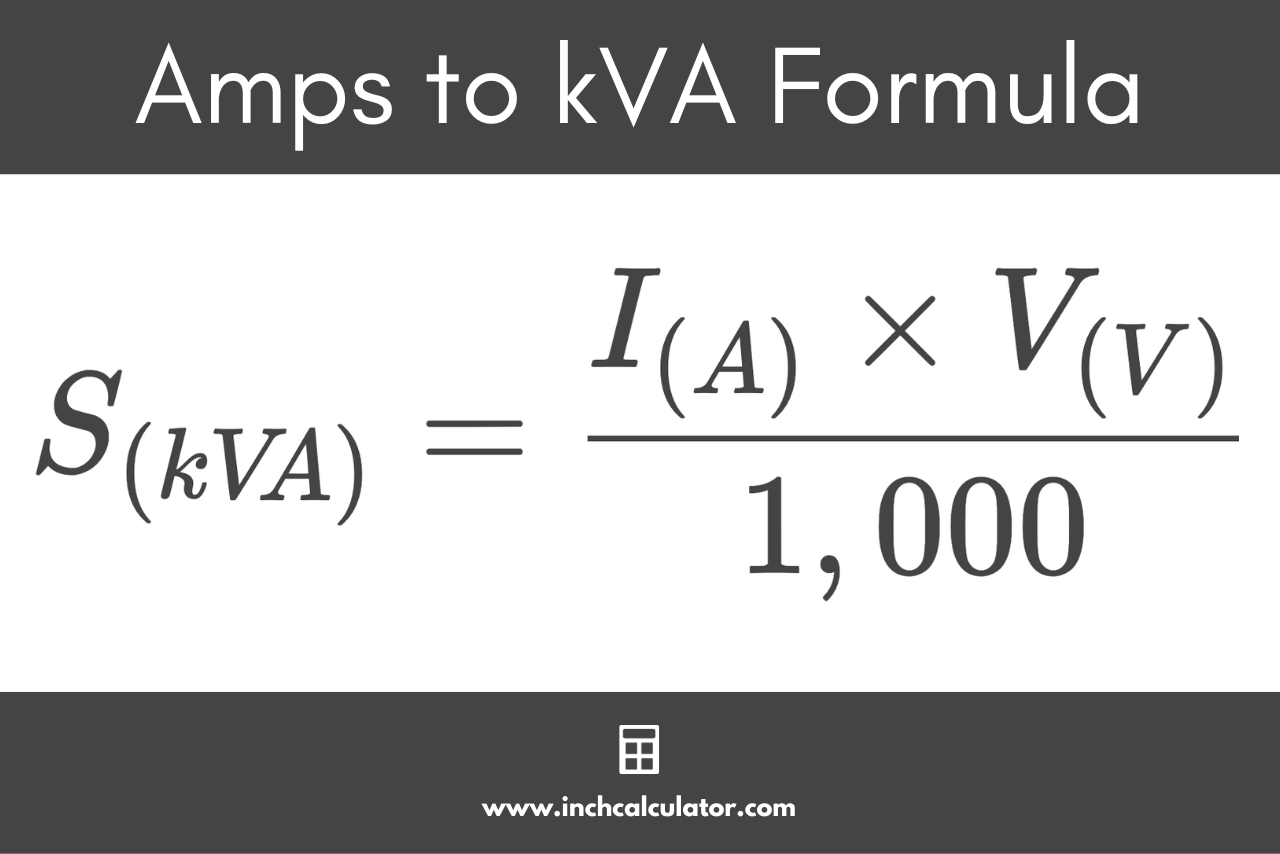# Amps to Kilovolt-Amps (kVA) Conversion Calculator

Convert amps (A) to kilovolt-amps (kVA) to find the power rating of an electrical system by entering the current in amps and voltage below. The calculator supports single-phase and three-phase systems.

amps
volts

## Kilovolt-Amps:

kVA
Learn how we calculated this below

## How to Convert Amps to kVA

Before we go over how to convert amps to kVA, we need to cover some basic terminology.

An Amp (A), short for Ampere, is a standard unit to measure the electrical current flowing in an electrical circuit. A current of 1A is equivalent to a rate of flow of charge of 1 coulomb in 1 second.

Power is a measure of the rate at which energy is transferred to devices connected to an electrical system.

Electrical power is typically measured in watts. However, in power systems, watts are only used to measure “real” power. More commonly, the power is measured in volt-amperes (VA) to show the total (apparent) power generated and delivered in power systems.

The relationship between Amps and kVA is well defined for single-phase and three-phase systems. These relationships are expressed through the following electrical power formulas.

### Amps to kVA Formula for Single-Phase Systems

Because amps and kVA are different things in an electrical circuit, the voltage is also needed for the conversion. By also using the voltage, it’s possible to convert amps to kVA using the Watt’s Law electrical power formula.

Using the electric power formula for a single phase system, the formula to convert amps to kVA can be derived:

S(kVA) = I(A) × V(V) / 1,000

Thus, apparent power S in kVA is equal to the current I in amps times the voltage V, divided by 1,000.Do you want to convert kVA to amps?

For example, let’s find the apparent power in kVA for 220-volt circuit with 40 amps of current.

S(kVA) = 40 A × 220 V / 1,000
S(kVA) = 8.8 kVA

### Amps to kVA Formula for Three-Phase Systems

Three-phase circuits require a slightly different formula for the conversion. To convert A to kVA in a three-phase circuit use the following formula.

S(kVA) = √3 × I(A) × V(V) / 1,000

For a three-phase system, the apparent power S in kVA is equal to the square root of 3 (1.732) times the current I in amps times volts V, divided by 1,000.

For example, find the apparent power in kVA for 440-volt three-phase circuit with 150 amps of current.

S(kVA) = 1.732 × 150 A × 440 V / 1,000
S(kVA) = 114.312 kVA

Be sure to check out our amps to volt-amps calculator for a similar conversion.